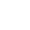﻿

/    2018-10-17

Python异常发生后，异常之后的代码就不执行了，对于这个事情的出现，Python程序员要进行异常处理!

```num1=input('>>: ') #输入一个字符串试试
int(num1)```

```num1=input('>>: ') #输入一个字符串试试
if num1.isdigit():
int(num1) #我们的正统程序放到了这里,其余的都属于异常处理范畴
elif num1.isspace():
print('输入的是空格,就执行我这里的逻辑')
elif len(num1) == 0:
print('输入的是空,就执行我这里的逻辑')
else:
print('其他情情况,执行我这里的逻辑')

'''

'''```

1.if判断式的异常处理只能针对某一段代码，对于不同的代码段的相同类型的错误你需要写重复的if来进行处理。

2.在你的程序中频繁的写与程序本身无关，与异常处理有关的if，会使得你的代码可读性极其的差

3.if是可以解决异常的，只是存在1,2的问题，所以，千万不要妄下定论if不能用来异常处理。

```def test():
print('test running')
choice_dic={
'1':test
}
while True:
choice=input('>>: ').strip()
if not choice or choice not in choice_dic:continue #这便是一种异常处理机制啊
choice_dic[choice]()```

python：为每一种异常定制了一个类型，然后提供了一种特定的语法结构用来进行异常处理

part1:基本语法

```try:
被检测的代码块
except 异常类型：
try中一旦检测到异常，就执行这个位置的逻辑```

part2:异常类只能用来处理指定的异常情况

```# 未捕获到异常，程序直接报错

s1 = 'hello'
try:
int(s1)
except IndexError as e:
print e```

part3:多分支

```s1 = 'hello'
try:
int(s1)
except IndexError as e:
print(e)
except KeyError as e:
print(e)
except ValueError as e:
print(e)```

part4:万能异常:Exception

```s1 = 'hello'
try:
int(s1)
except Exception as e:
print(e)```

1.如果你想要的效果是，无论出现什么异常，我们统一丢弃，或者使用同一段代码逻辑去处理他们，那么，大胆的去做吧，只有一个Exception就足够了。

Exception

```s1 = 'hello'
try:
int(s1)
except Exception,e:
'丢弃或者执行其他逻辑'
print(e)

#如果你统一用Exception，没错，是可以捕捉所有异常，但意味着你在处理所有异常时都使用同一个逻辑去处理（这里说的逻辑即当前expect下面跟的代码块）```

2.如果你想要的效果是，对于不同的异常我们需要定制不同的处理逻辑，那就需要用到多分支了。

```s1 = 'hello'
try:
int(s1)
except IndexError as e:
print(e)
except KeyError as e:
print(e)
except ValueError as e:
print(e)```

```s1 = 'hello'
try:
int(s1)
except IndexError as e:
print(e)
except KeyError as e:
print(e)
except ValueError as e:
print(e)
except Exception as e:
print(e)```

part5:异常的其他机构

```s1 = 'hello'
try:
int(s1)
except IndexError as e:
print(e)
except KeyError as e:
print(e)
except ValueError as e:
print(e)
#except Exception as e:
#    print(e)
else:
print('try内代码块没有异常则执行我')
finally:
print('无论异常与否,都会执行该模块,通常是进行清理工作')```

part6:主动触发异常

```try:
raise TypeError('类型错误')
except Exception as e:
print(e)```

part7:自定义异常

```class EvaException(BaseException):
def __init__(self,msg):
self.msg=msg
def __str__(self):
return self.msg

try:
raise EvaException('类型错误')
except EvaException as e:
print(e)```

part8:断言

```# assert 条件
assert 1 == 1
assert 1 == 2```

part9：try..except的方式比较if的方式的好处

try..except这种异常处理机制就是取代if那种方式，让你的程序在不牺牲可读性的前提下增强健壮性和容错性

1：把错误处理和真正的工作分开来

2：代码更易组织，更清晰，复杂的工作任务更容易实现;

3：毫无疑问，更安全了，不至于由于一些小的疏忽而使程序意外崩溃了;

try...except虽然好用，但应尽量少用，因为它本身就是你附加给你的程序的一种异常处理的逻辑，与你的主要的工作是没有关系的，这种东西加的多了，会导致你的代码可读性变差，只有在有些异常无法预知的情况下，才应该加上try...except，其他的逻辑错误应该尽量修正。(8)

﻿